Question

Given the following array of integers (of capacity 20) with 12 items:

0 1 2 3 4 5 6 7 8 9 10 11 12 13 14 15 16 17 18 19

 8 4 10 15 5 7 11 3 9 13 1 6

Index of last element = 11

Does this array represent a min heap? If not, convert it to a min heap (i.e., “heapify” it). Please show all steps.

The original array given is like:

8,4,10,15,5,7,11,3,9,13,1,6

The index of the last element is 11.

The array does not represent the min heap since the minimum element is not at the top.

We can convert the above array to the min heap to the below array:

1,3,6,5,4,7,11,15,9,13,8,10

We can build the min heap tree by converting the tree into min heap: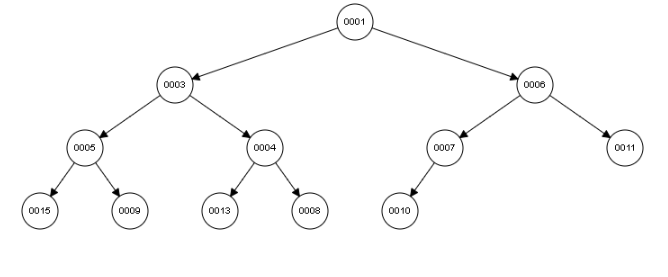**Please Hit Like if you appreciate my answer. For further doubts on the or answer please drop a comment, I'll be happy to help. Thanks for posting.**

#### Earn Coins

Coins can be redeemed for fabulous gifts.

Similar Homework Help Questions
• ### Tree & Hash Table & Heap Use the following integer keys 73, 58, 91, 42, 60, 130, 64, 87 to perform the followings: a) Binary Search Tree - Draw a binary search tree - Retrieve the integers ke...

Tree & Hash Table & Heap Use the following integer keys 73, 58, 91, 42, 60, 130, 64, 87 to perform the followings: a) Binary Search Tree - Draw a binary search tree - Retrieve the integers keys in post-order - Retrieve the integers keys in pre-order - Draw a binary search tree after node 58 is deleted b) Create a Hash Table using the methods described below. Show the final array after all integer keys are inserted. Assumes that...

• ### Write a program that asks the user to input 10 integers of an array. The program...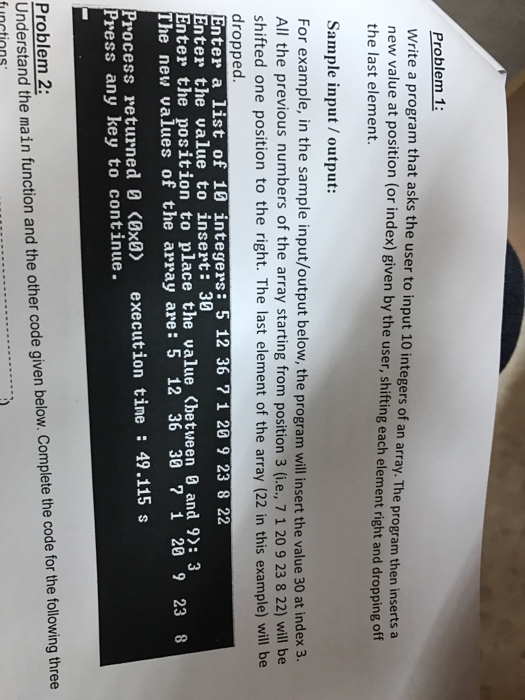Write a program that asks the user to input 10 integers of an array. The program then inserts a new value at position (or index) given by the user, shifting each element right and dropping off the last element. For example, in the sample input/output below, the program will insert the value 30 at index 3. All the previous numbers of the array starting from position 3 (i.e., 7 1 20 9 23 8 22 will be shifted one position...

• ### You are given an array containing integers in the sorted order: [O]    ...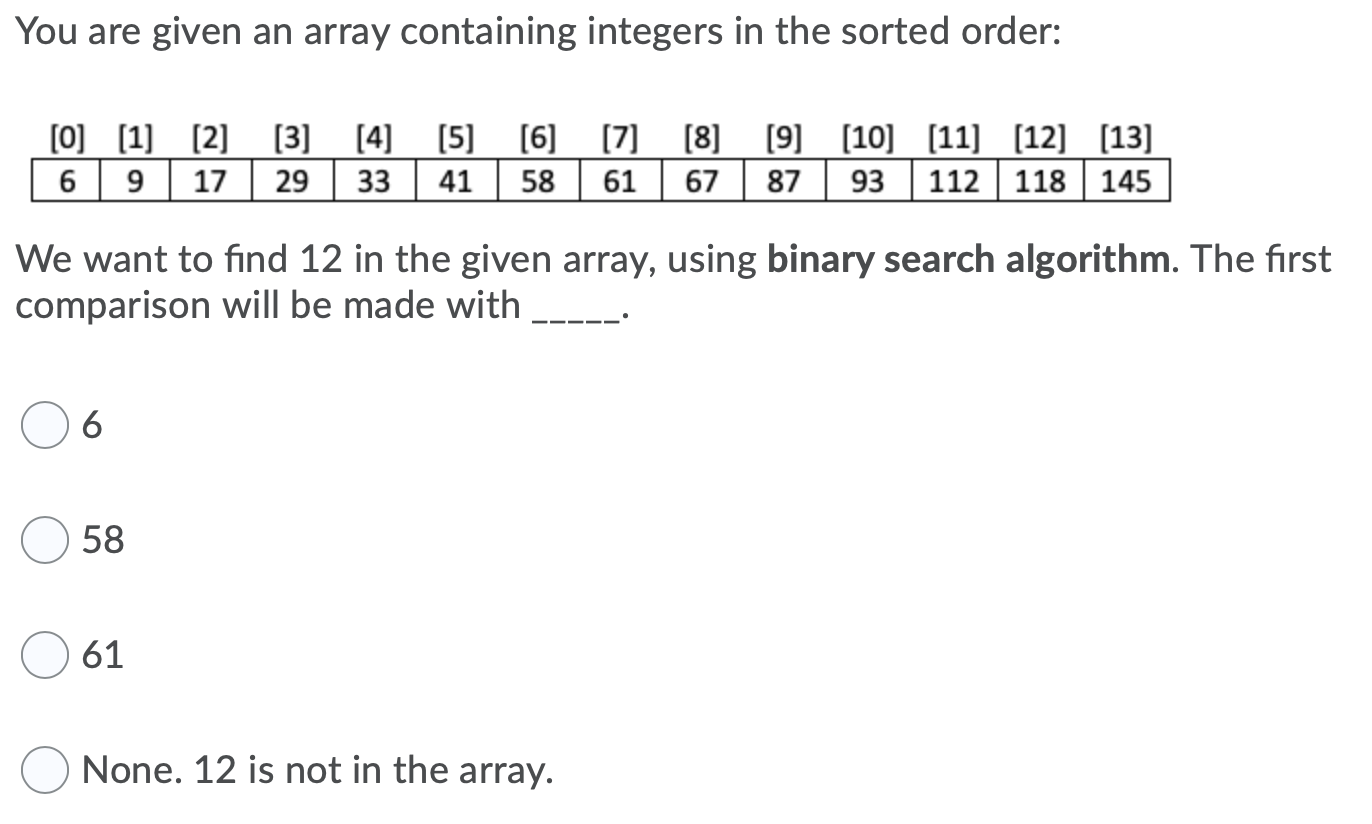You are given an array containing integers in the sorted order: [O]        6 9 17 29 33 41 58 61       67 87 93 112 118 145 We want to find 12 in the given array, using binary search algorithm. The first comparison will be made with ----- 6 58 61 None. 12 is not in the array.

• ### Tree & Hash Table & Heap Use the following integer keys 73, 58, 91, 42, 60,...

Tree & Hash Table & Heap Use the following integer keys 73, 58, 91, 42, 60, 130, 64, 87 to perform the followings: a) Binary Search Tree - Draw a binary search tree - Retrieve the integers keys in post-order - Retrieve the integers keys in pre-order - Draw a binary search tree after node 58 is deleted b) Create a Hash Table using the methods described below. Show the final array after all integer keys are inserted. Assumes that...

• ### Write a C++ function, smallestIndex, that takes as parameters an int array and its size and...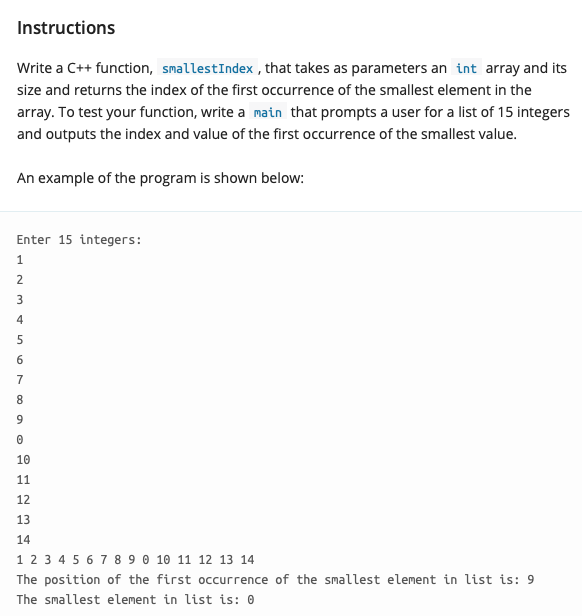Write a C++ function, smallestIndex, that takes as parameters an int array and its size and returns the index of the first occurrence of the smallest element in the array. To test your function, write a main that prompts a user for a list of 15 integers and outputs the index and value of the first occurrence of the smallest value. The program should print out Enter 15 integers: The position of the first occurrence of the smallest element in...

• ### Declare an array of integers called ages The arra bold 119 integers Prompt and get an...Declare an array of integers called ages The arra bold 119 integers Prompt and get an ange from the user for the fourth element in the artay Prompt and get a score from the user for the last element in the array HTML tau nt ages(11% printf Enter a number for the fourth dlement; scan%,age30 rints Enter a number for the last element, scant, r. ages(119D 5 6 7 8 9 ERT 7 Y U O P F G HUKL

• ### 14 18 11 10 19 16 23 28 15 21 Again, recall that in a Java...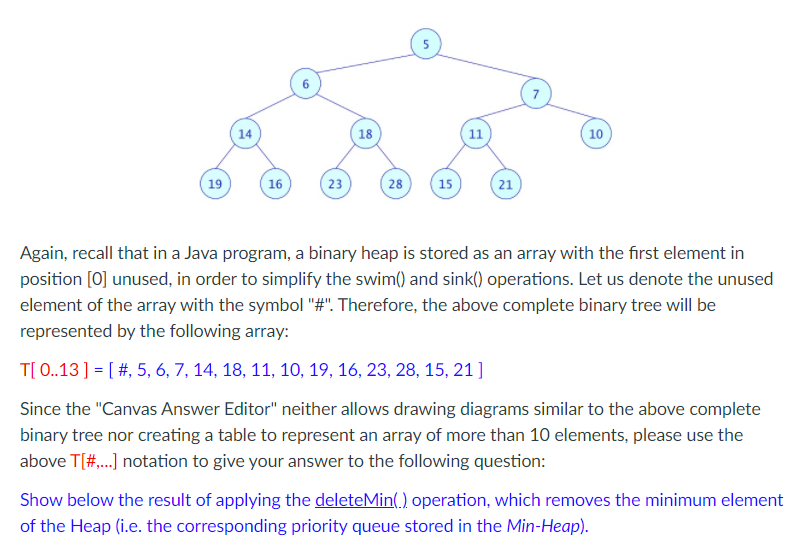14 18 11 10 19 16 23 28 15 21 Again, recall that in a Java program, a binary heap is stored as an array with the first element in position [O] unused, in order to simplify the swim() and sink() operations. Let us denote the unused element of the array with the symbol "#". Therefore, the above complete binary tree will be represented by the following array: T[ 0..13 ] = [ #, 5, 6, 7, 14, 18, 11,...

• ### (20 points) You are given an array A of distinct integers of size n. The sequence...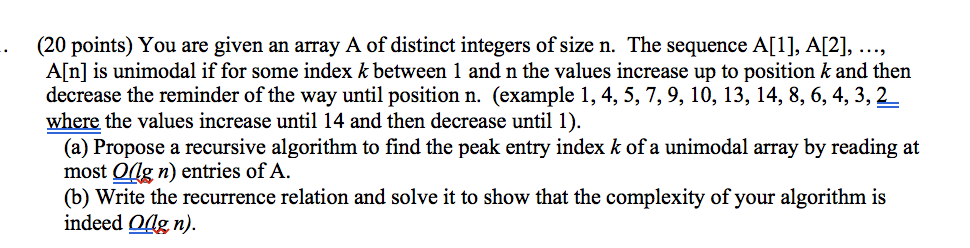(20 points) You are given an array A of distinct integers of size n. The sequence A, A, ..., A[n] is unimodal if for some index k between 1 and n the values increase up to position k and then decrease the reminder of the way until position n. (example 1, 4, 5, 7, 9, 10, 13, 14, 8, 6, 4, 3, 2 where the values increase until 14 and then decrease until 1). (a) Propose a recursive algorithm to...

• ### 5. A three-heap with n elements can be stored in an array A, where A[O] contains...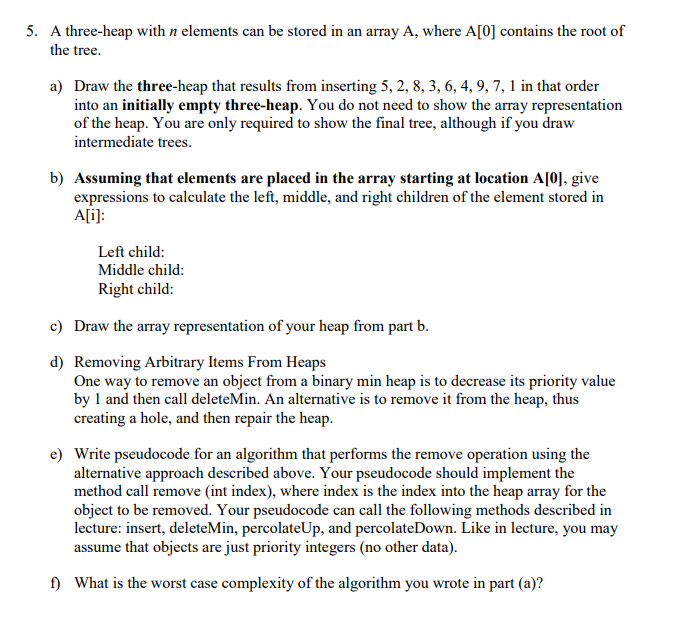5. A three-heap with n elements can be stored in an array A, where A[O] contains the root of the tree. a) Draw the three-heap that results from inserting 5, 2, 8, 3, 6, 4, 9, 7, 1 in that order into an initially empty three-heap. You do not need to show the array representation of the heap. You are only required to show the final tree, although if you draw intermediate trees. b) Assuming that elements are placed in...

• ### Please ignore red marks. Thanks 6. (8 pts) Illustrate the algorithmic operations on the maximum binary...Please ignore red marks. Thanks 6. (8 pts) Illustrate the algorithmic operations on the maximum binary heap data sti 'perations on the maximum binary heap data structure as directed. BUILD-MAX-HEAP(A) MAX-HEAPIFY (A. i) 1 A heap-size = A.length 11 = LEFT() 2 for i = A.length/2) downto 1 2 r = RIGHT() 3 MAX-HEAPIFY (A,i) 3 if / S 4.heap-size and All > A[i] HEAP-EXTRACT-MAX (A) 4 largest = 1 5 else largest = 1 1 if A.heap-size <1 6...